# Archived: Proposed guidelines: reducing nitrogen oxide emissions from stationary combustion turbines: appendix 1

## Appendix 1: Quantification Protocol

### A. Conformance Determination: Testing Methods

1. Conformance of a new combustion turbine with these Guidelines should be determined using either of the following methods:
1. the output-based method (expressed as NO2/GJ); or
2. the concentration-based method (ppmv NOx) at 15% O2, on a dry basis.

#### Output-based Method

1. For a combustion turbine to meet the applicable emission limit set out in Table 1, at the operating conditions stated in Part D, the operator should conform with the following principles to calculate NOx emission rates:
1. Measure concurrently the following:
1. the stack gas flow as per U.S. Environmental Protection Agency (EPA) method entitled Method 2 - Determination of Stack Gas Velocity and Volumetric Flow Rate (Type S pitot tube) set out in Appendix A-1 to part 60 of the CFR or the method published by Environment and Climate Change Canada known as Method B: Determination of Stack Gas Velocity and Volumetric Flow Rate (EPS 1/RM/8), and
2. the NOx concentration in accordance with the EPA method entitled Method 7E - Determination of Nitrogen Oxides Emissions from Stationary Sources (Instrumental Analyzer Procedure), set out in Appendix A-4 to Part 60 of the CFR, to determine the NOx emission rate during three contiguous thirty-minute periods. Non-ideal stack gas flow conditions may require the application of EPA Methods 2G or 2F.
2. Non-ideal stack gas flow conditions may require the application of EPA Methodsmethod entitled Method 2G - Determination of Stack Gas Velocity and Volumetric Flow Rate with Two-dimensional Probes, set out in Appendix A-2 to part 60 of the CFR or EPA method entitled Method 2F - Determination of Stack Gas Velocity and Volumetric Flow Rate with Three-dimensional Probes, set out in Appendix A-1 to part 60 of the CFR.

3. Calculate the NOx emission rate using Equation (1) below:

(1)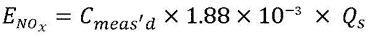Long description of Equation 1

The emission rate for nitrogen oxide, in grams per hour, equals the measured NOx concentration in parts per million on a dry basis, multiplied by one point eight eight times ten to the minus three, multiplied by the stack gas flow rate in cubic meters per hour on a dry basis at the reference conditions of 25 degree Celsius and 101.325 kilopascals.

where:

ENOx is the emission rate expressed as g NO2/h

Cmeas'd is the NOx concentration, in ppmv, on a dry basis

1.88 × 10-3 is the conversion factor for NOx from ppmv to g/m³

Qs is the stack gas flow rate, in m³/hr, on a dry basis and in reference conditions of 25°C and 101.325 kPa

4. Alternately, determine the NOx emission rate by measuring the heat (fuel) input and NOx and O2 concentration in accordance with the EPA method entitled Method 20 - Determination of Nitrogen Oxides, Sulfur Dioxide, and Diluent Emissions from Stationary Gas Turbines, set out in Appendix A-7 to part 60 of the CFR, during three contiguous thirty-minute periods. The NOxemission rate is calculated using Equation (2) below:

(2)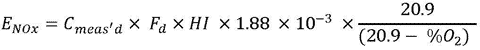Long description of Equation 2

The emission rate for nitrogen oxide, in grams per hour, equals the measured NOx concentration in parts per million on a dry basis, multiplied by the F-factor for natural gas from Appendix A of Report EPS 1/PG/7 (revised), multiplied by the gross heat input of the natural gas turbine system in gigajoules per hour, multiplied by one point eight eight times ten to the minus three, multiplied by the ratio of twenty point nine to the difference between twenty point nine and the stack gas oxygen concentration on a dry basis in percent.

where:

ENOx is the NOxemission rate expressed as g NO2/h

Cmeas'd is the NOx concentration, in ppmv, on a dry basis

Fd is the F-factor for natural gas on a dry basis, 240 DSm³/GJ, mentioned in Appendix A, Table A-1 of the method published by Environment and Climate Change Canada entitled Protocols and Performance Specifications for Continuous Monitoring of Gaseous Emissions from Thermal Power Generation, (EPS 1/PG/7)

1.88 × 10-3 is the conversion factor for NOx from ppmv to g/m³

HI is the gross heat input to the turbine system (natural gas), in GJ/h

%O2 is the measured O2concentration, in % (v/v), on a dry basis

5. Verify conformance of the combustion turbine not operating in a cogeneration application with the applicable output-based limit according to the Equation (3) below:

(3)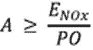Long description of Equation 3

The applicable output-based emission limit from Table 1 of these Guidelines, in grams per gigajoule, should be greater than or equal to the ratio of the emission rate for nitrogen oxide, as calculated using Equations 1 or equation2, to the power output as electricity and/or shaft power produced by the combustion turbine during an emission testing period in gigajoules per hour.

where:

A is the applicable output-based emission limit in Table 1, in g/GJ

ENOx is the NOxemission rate, in g NO2/h, calculated using equations (1) or equation(2)

PO is the power output, the combined electricity and shaft power produced by the combustion turbine and the steam turbine(s) during an emission testing period, in GJ/h

6. In the case of a combustion turbine operating in a cogeneration application, verify conformance with the applicable output-based limit by calculating the NOx emission rate according to the Equation (4) below:

(4)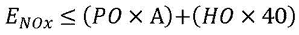Long description of Equation 4

For a combustion turbine operating in a cogeneration application, the emission rate for nitrogen oxide, in grams per hour, as calculated using Equations 1or Equation2, must be less than or equal to the sum of the power output as electricity and/or shaft power, multiplied by the applicable output-based emission limit from Table 1 of the Guidelines, in grams per gigajoule, plus the heat output (normally steam), produced by the combustion turbine during an emission testing period in gigajoules per hour, multiplied by the cogeneration coefficient of 40 grams per gigajoule.

where:

ENOx is the NOxemission rate, in g NO2/h, as calculated using equations (1) or equation(2)

PO is the power output, the combined electricity and shaft power, produced by the combustion turbine and the steam turbine(s) during an emission testing period, in GJ/h

A is the applicable output-based emission limit in Table 1, in g/GJ

HO is the heat output (normally steam) produced by the combustion turbine operating in cogeneration application during an emission testing period, in GJ/h

40 is the Cogeneration Coefficient, in g/GJ

#### Concentration-based Method

1. For a combustion turbine to meet the concentration-based emission limits set out in Table 2 at the operating conditions outlined in Part D, the operator should conform with the following principles:
1. Measure concurrently the O2 and NOxconcentrations in accordance with the EPA method entitled Method 20 - Determination of Nitrogen Oxides, Sulfur Dioxide, and Diluent Emissions from Stationary Gas Turbines, set out in Appendix A-7 to part 60 of the CFR, during three contiguous thirty-minute periods.

2. Correct each measured NOx value to a concentration at 15% oxygen using Equation (5) below:

(5)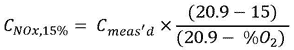Long description of Equation 5

In order to conform to the concentration-based emission limit set out in Table 2 of the Guidelines, determine the NOxconcentration in parts per million on a dry basis, corrected to 15% O2, which equals the measured NOxconcentration, multiplied by the ratio of the difference between twenty point nine and fifteen to the difference between twenty point nine and the measured stack gas oxygen concentration on a dry basis in percent. Then verify conformance using Equation 6.

where:

CNOx,15% is the concentration of NOx corrected to 15% O2, in ppmv, on a dry basis

Cmeas'd is the measured NOxconcentration, in ppmv, on a dry basis

%O2 is the measured O2concentration, in % (v/v), on a dry basis

3. Calculate the final NOx concentration in ppmv at 15% O2, on a dry basis by averaging the value for each thirty-minute period.

4. Verify conformance of the combustion turbine with the applicable concentration-based emission limit according to Equation (6) below:

(6)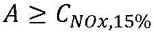Long description of Equation 6

The NOx concentration calculated from Equation 5 should be less than or equal to the applicable output-based emission limit from Table 2 of the Guidelines, in parts per million on a dry basis.

where:

A is the concentration-based emission limit in Table 2, in ppmv

CNOx,15% is the concentration of NOx corrected to 15% O2, in ppmv, on a dry basis calculated using equation (5)

### B. Quantification of Production

1. The operator of the combustion turbine should install, maintain and operate a device to measure the mechanical output and, as the case may be, the gross electrical output of the combustion turbine during NOx testing. The combined values from these devices should give the power (mechanical and/or electric) output of the unit.

2. The operator of the combustion turbine should install, maintain and operate a device to measure the heat output (normally steam) delivered of the combustion turbine operating in a cogeneration application during NOx testing.

### C. Conformance Determination: Continuous Emissions Monitoring System

1. A Continuous Emissions Monitoring System (CEMS) may be used to measure NOx emissions in lieu of annual stack testing.

2. A CEMS should be used on non-peaking electricity generating combustion turbines with a power rating greater than 25 MW.

3. The CEMS should meet the specifications for design, installation, certification and quality assurance/quality control (QA/QC) set out in the method published by Environment and Climate Change Canada entitled Protocols and Performance Specifications for Continuous Monitoring of Gaseous Emissions from Thermal Power Generation, (EPS 1/PG/7) or the method published by Alberta Environmental Protection entitled Continuous Emission Monitoring System (CEMS) Code.

4. As an alternative, a Predictive Emission Monitoring System (PEMS) is an acceptable equivalent to a CEMS if it meets the EPA method entitle Method 16 - Specifications and Test Procedures for Predictive Emission Monitoring Systems in Stationary Sources set out in the Title 40, Chapter I, Subchapter C, part 60, of the CFR.

5. NOx emissions information (e.g., data averages) should be collected for the purpose of these Guidelines when the turbine operates at the conditions outlined in Part D.

6. For the output-based method, the operator should also, during the period of data collection:
1. Install, maintain and operate a device to measure heat input so that NOx emissions information can be converted to mass per hour emission rate using equation B-1 in Appendix B of the method published by Environment and Climate Change Canada entitled Protocols and Performance Specifications for Continuous Monitoring of Gaseous Emissions from Thermal Power Generation, (EPS 1/PG/7). An average rate should be determined.

2. IInstall, maintain and operate a device to continuously measure the mechanical and, as the case may be, the electrical output of the unit; determine the combined mechanical and electric output of the unit; calculate the average hourly output.

3. Measure the heat output (normally steam) delivered from the combustion turbine operating in a cogeneration application during an emissions testing period; calculate the average hourly output.

4. Verify conformance with the applicable output based limit by calculating the emission rate using Equations (3) and equation(4) in Part A.

### D. Operating Conditions

1. The measurement of NOx emissions should be done under normal operating conditions as follows:
1. All emission performance tests should be conducted when the combustion turbines is operated at a load level that is within 70% to 100% of its power rating.

2. Despite paragraph (a), testing may be conducted at the highest achievable load, if it is not practically possible to operate the combustion turbine at a load equal to or greater than 70% of its power rating.

3. Testing should reflect typical operation conditions and fuel characteristics. Testing under atypical or artificial conditions will not satisfy the requirements of these guidelines.

4. Testing should be done at ambient temperatures greater than or equal to -18°C.

2. When more than one combustion turbine is venting through a common stack, the emission samples taken from that stack should take place with one combustion turbine operating at a time.

However, if more than one combustion turbine shares air emission control equipment, one emission sample can be taken for all turbines when taken downstream of the air emission control equipment.

### E. Accuracy of Data

1. Measurement devices required for these Guidelines should be calibrated at the most frequent of the following:
1. at least once every three calendar years;

2. at the frequency recommended by the manufacturer; or

3. as specified in the test methods for the devices.
2. Each measurement device should enable measurements to be made within an accuracy of ± 5%.